# sin cos tan

sin cos tan

In this mini-lesson, we will explore the trigonometric ratios sin, cos, and tan.

Trigonometry is the study of the relationship between the angles and sides of a triangle (especially of a right-angled triangle).

Pythagoras theorem and Trigonometry were the most important concepts in the construction of the Pyramids.Pythagoras worked on the relationship between the sides of a right triangle whereas the people like Hipparcus worked on the relationship between the sides and angles of a right triangle.

Sin, cos, and tan are the primary trigonometric ratios and they are the basics of trigonometry.

Let's study in detail about sin, cos, and tan.

## Lesson Plan

 1 What Do We Mean By Sin Cos Tan? 2 Important Notes on Sin Cos Tan 3 Tips and Tricks on Sin Cos Tan 4 Solved Examples 5 Interactive Questions

## What Do We Mean By Sin Cos Tan?

Sin, cos, and tan are the three primary trigonometric ratios where each of which gives the ratio of two (of three) sides of a right-angled triangle.

We know that the longest side of a right-angled triangle is known as the "hypotenuse" and the other two sides are known as the "legs."

In trigonometry, the longest side of a right-angled triangle is still known as the "hypotenuse" but the other two legs are named to be:

• opposite side and
• adjacent side

We decide the "opposite" and "adjacent" sides based upon the angle which we are talking about.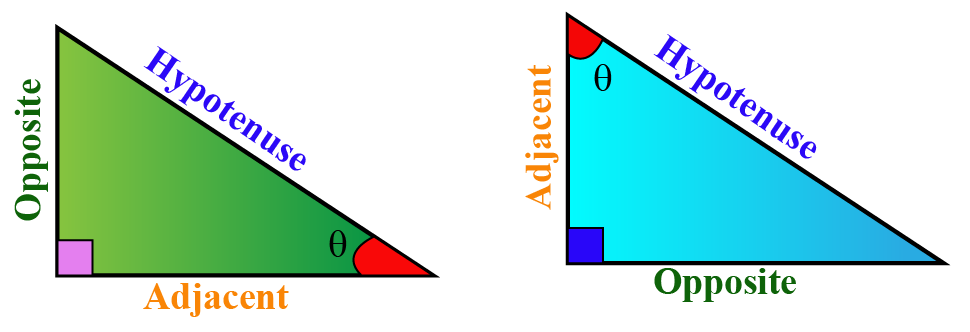## What Are the General Formulas of Sin Cos Tan?

Sin, cos, and tan are defined in terms of two of the three sides (opposite, adjacent, and hypotenuse) of a right-angled triangle.

### Formulas

Here are the formulas of sin, cos, and tan.

\begin{align} \sin \theta &= \dfrac{ \text{Opposite}}{ \text{Hypotenuse}}\\[0.2cm] \cos\theta &= \dfrac{ \text{Adjancent}}{ \text{Hypotenuse}}\\[0.2cm] \tan\theta &= \dfrac{ \text{Opposite}}{ \text{Adjacent}}\\[0.2cm] \end{align}

Apart from these three trigonometric ratios, we have another three ratios called csc, sec, and cot which are the reciprocals of sin, cos, and tan respectively.

Example

Find the sin, cos, and tan of the following triangle for the given angle $$\theta$$.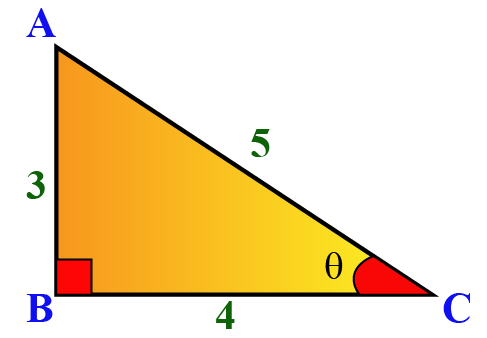Solution

In the triangle, the longest side (or) the side opposite to the right angle is $$AC$$ and hence $$AC$$ is the hypotenuse.

The angle opposite to $$\theta$$ is $$AB$$ and so $$AB$$ is the opposite side.

The angle adjacent to $$\theta$$ is $$BC$$ and so $$BC$$ is the adjacent side.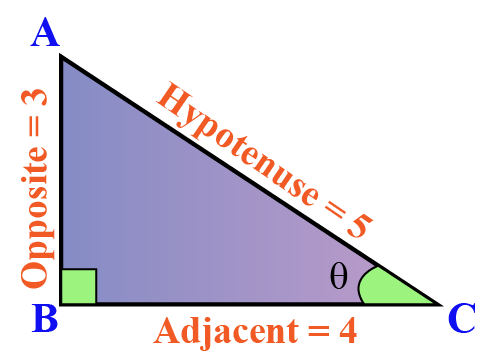Now we find $$\sin \theta, \cos \theta,$$ and $$\tan \theta$$ using the above formulas:

\begin{align} \sin \theta &= \dfrac{ \text{Opposite}}{ \text{Hypotenuse}}= \dfrac{AB}{AC}= \dfrac{3}{5}\\[0.2cm] \cos\theta &= \dfrac{ \text{Adjancent}}{ \text{Hypotenuse}}=\dfrac{BC}{AC}=\dfrac{4}{5}\\[0.2cm] \tan\theta &= \dfrac{ \text{Opposite}}{ \text{Adjacent}}=\dfrac{AB}{BC}=\dfrac{3}{4}\\[0.2cm] \end{align}Tips and Tricks
1. Are you struggling to remember these formulas? Here is a trick to remember the formulas of sin, cos, and tan.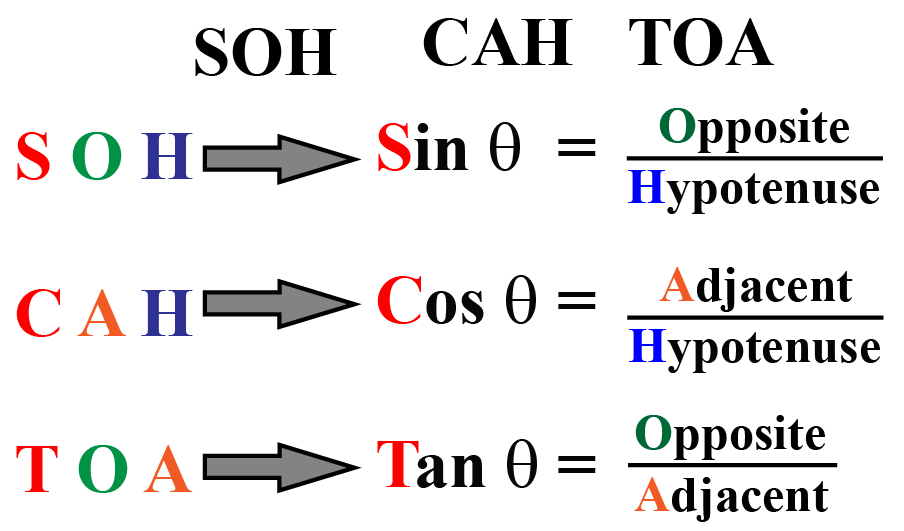## Sin Cos Tan Chart

Remember that the trigonometric ratios do not depend upon the side lengths of the triangle but rather they depend upon the angle because ultimately, we are taking the ratio of the sides.

Here is an illustration where you can change the angle using the given slider; and where you can change the side lengths by dragging the point B.

You can still see that the values of the trigonometric ratios stay constant at a particular angle though the side lengths are being changed.

We can find the sin, cos, and tan of any angle by using a graphing calculator using the following buttons and by setting the angle to degrees/radians as required.### Sin Cos Tan Calculator

Here is the "Sin Cos Tan Calculator."

You can enter any angle in degrees/radians and select any trigonometric function.

It will show the value of the selected trigonometric function with respect to the given angle.

### Sin Cos Tan Chart

Though we can find the values of cos, sin, and tan using the calculator, there is a chart with some standard angles 0o, 30o, 45o, 60o, and 90o.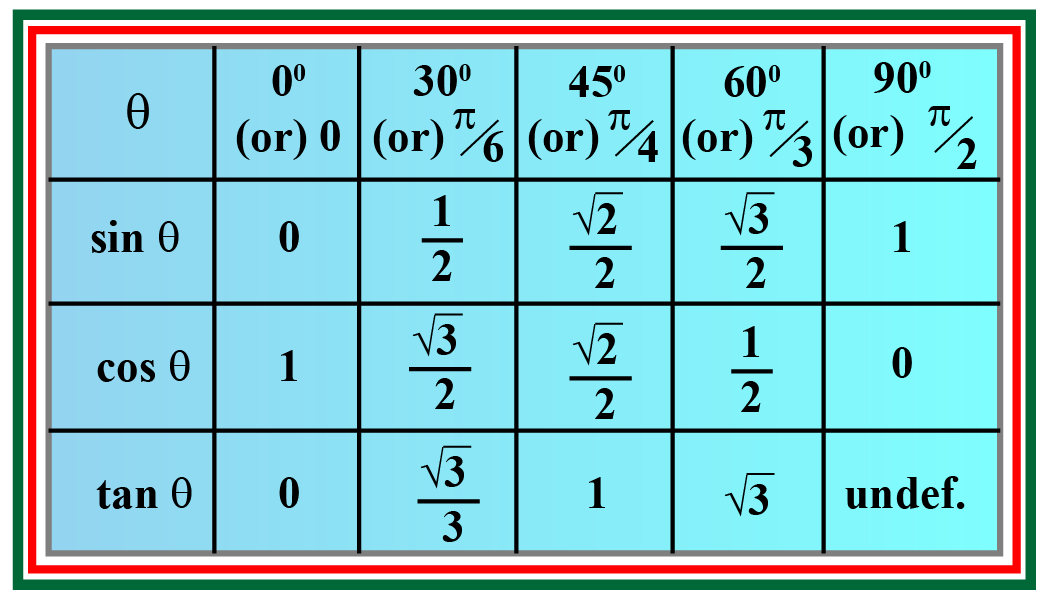Tips to remember sin cos tan table:

The things that you need to memorize from this chart are:

• The angles  0o, 30o, 45o, 60o, and 90 in order.
• The first row (of sin) can be remembered like this: $$\dfrac{\sqrt 0}{2}, \dfrac{\sqrt 1}{2}, \dfrac{\sqrt 2}{2}, \dfrac{\sqrt 3}{2},$$ and $$\dfrac{\sqrt 4}{2}$$

That's all you need to remember because:

• The row of cos is as same as the row of sin just in the reverse order.
• Each value in the row of tan is obtained by dividing the corresponding values of sin by cos because tan = sin/cos.

You can see how is tan = sin/cos here:

\begin{align}\dfrac{\sin \theta}{\cos \theta}&= \dfrac{ \text{Opposite}}{ \text{Hypotenuse}} \div \dfrac{ \text{Adjacent}}{ \text{Hypotenuse}}\\[0.2cm] &= \dfrac{ \text{Opposite}}{ \text{Hypotenuse}} \times \dfrac{ \text{Hypotenuse}}{\text{Adjacent}}\\[0.2cm] &= \dfrac{ \text{Opposite}}{ \text{Adjacent}}\\[0.2cm] &= \tan \theta \end{align}

## Where Do We Use Sin Cos Tan in Real Life?

We do use sin, cos, and tan to solve many real-life problems. Here is an example.

Example

A ladder leans against a brick wall making an angle of 50o with the horizontal.

If the ladder is at a distance of 10 ft from the wall, then up to what height of the wall the ladder reaches?

Solution

Let us assume that the ladder reaches till $$x$$ ft of the wall.

Using the given information: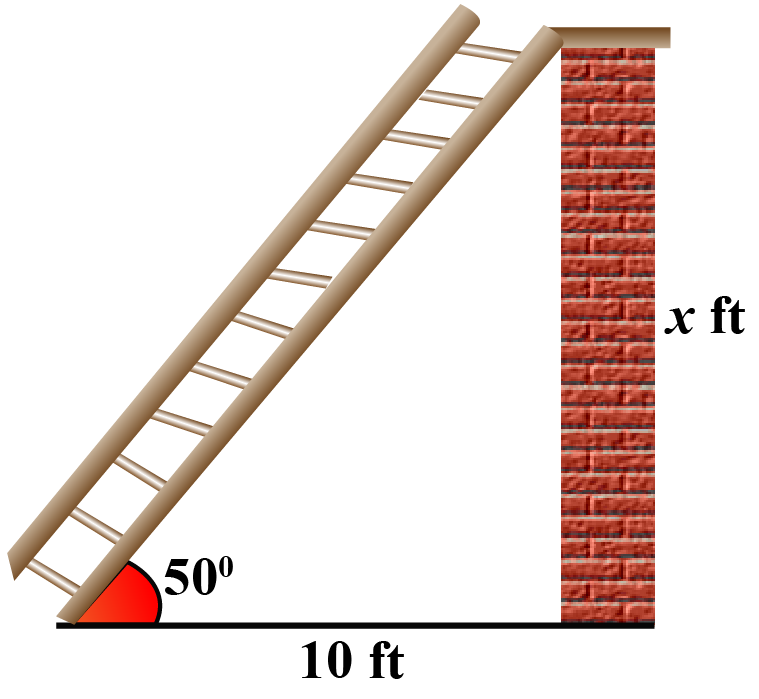Here, we know the adjacent side (which is 10 ft) and we have to find the opposite side (which is $$x$$ ft).

So we use the relation between the opposite and the adjacent sides which is tan.

\begin{align} \tan 50^\circ &= \dfrac{x}{10}\\[0.2cm] x&= 10 \tan 50^\circ\\[0.2cm] x&\approx 11.9 \text{ ft} \end{align}

Here, tan 50o is calculated using the calculator and the final answer is rounded up to 1 decimal.

Therefore, the ladder reaches up to 11.9 ft of the wall.

## Solved Examples

 Example 1

Can we help Rachel find $$\cos \theta$$ with respect to the following triangle?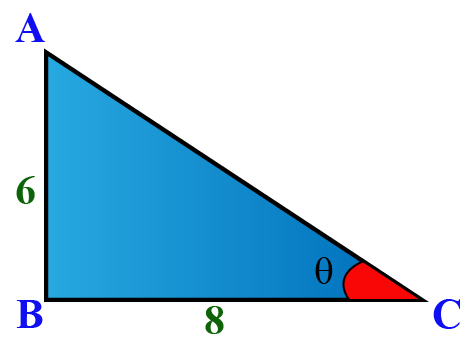Solution

To find $$\cos \theta$$, we need the adjacent side and the hypotenuse.

Here, the adjacent side is, $$BC=8$$

But we are not given the hypotenuse, $$AC$$.

To find this, we use Pythagoras theorem:

\begin{align}AC^2 &=AB^2+BC^2\\[0.2cm] &=6^2+8^2\\[0.2cm] &=100\\[0.2cm] AC&= \sqrt{100}=10 \end{align}

Therefore, $\cos \theta = \dfrac{ \text{Adjacent}}{ \text{Hypotenuse}}= \dfrac{8}{10}= \dfrac{4}{5}$

 $$\cos \theta=\dfrac{4}{5}$$
 Example 2

Find the exact length of the shadow cast by a 15 ft lamp post when the angle of elevation of the sun is 60º.

Solution

Let us assume that the length of the shadow of the lamp post is $$x$$ ft.

Using the given information: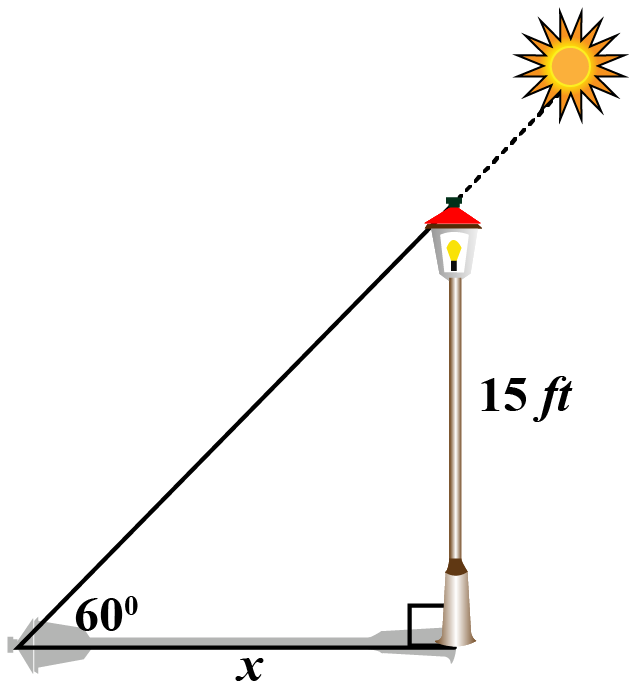Applying tan to the given triangle

\begin{align} \tan 60^\circ &= \dfrac{15}{x}\\[0.2cm] x&= \dfrac{15}{\tan 60^\circ}\\[0.2cm] x &= \dfrac{15}{\sqrt 3} \,\,\, \text{(Using chart)} \\[0.2cm] x&= \dfrac{15 \sqrt 3}{3}\end{align}

 $$\therefore$$ The length of the shadow of the lamp post is $$\dfrac{15 \sqrt 3}{3}$$ ft

## Interactive Questions

Here are a few activities for you to practice.

Select/type your answer and click the "Check Answer" button to see the result.

## Let's Summarize

The mini-lesson targeted the fascinating concept of sin cos tan. The math journey around sin cos tan starts with what a student already knows, and goes on to creatively crafting a fresh concept in the young minds. Done in a way that not only it is relatable and easy to grasp, but also will stay with them forever. Here lies the magic with Cuemath.

## About Cuemath

At Cuemath, our team of math experts is dedicated to making learning fun for our favorite readers, the students!

Through an interactive and engaging learning-teaching-learning approach, the teachers explore all angles of a topic.

Be it worksheets, online classes, doubt sessions, or any other form of relation, it’s the logical thinking and smart learning approach that we, at Cuemath, believe in.

## 1. How to use sin cos tan?

We can use sin cos and tan to solve real-world problems.

To solve any problem, we first draw the figure that describes the problem and we use the respective trigonometric ratio to solve the problem.

## 2. How to find sin cos tan?

To find sin, cos, and tan we use the following formulas:

\begin{align} \sin \theta &= \dfrac{ \text{Opposite}}{ \text{Hypotenuse}}\\[0.2cm] \cos\theta &= \dfrac{ \text{Adjancent}}{ \text{Hypotenuse}}\\[0.2cm] \tan\theta &= \dfrac{ \text{Opposite}}{ \text{Adjacent}}\\[0.2cm] \end{align}

For finding sin, cos, and tan of standard angles, you can use the chart mentioned in this page.

To find sin, cos, and tan of any angle, you can use the calculator in this page (or) a graphing calculator.

## 3. How to do sin cos tan?

To find sin, cos, and tan we use the following formulas:

\begin{align} \sin \theta &= \dfrac{ \text{Opposite}}{ \text{Hypotenuse}}\\[0.2cm] \cos\theta &= \dfrac{ \text{Adjancent}}{ \text{Hypotenuse}}\\[0.2cm] \tan\theta &= \dfrac{ \text{Opposite}}{ \text{Adjacent}}\\[0.2cm] \end{align}

For finding sin, cos, and tan of standard angles, you can use the chart mentioned in this page.

To find sin, cos, and tan of any angle, you can use the calculator in this page (or) a graphing calculator.

## 4. If sin θ = 2 over 3 and tan θ < 0, what is the value of cos θ?

It is given that $$\sin \theta$$ is positive and $$\tan \theta$$ is negative.

So $$\theta$$ must be in the Quadrant II, where $$\cos \theta$$ is negative.

Now, $$\sin \theta= \dfrac{2}{3}= \dfrac{ \text{Opposite}}{ \text{Hypotense}}$$.

So we can assume that

\begin{align} \text{Opposite }&=2k\\ \text{Hypotenuse }&=3k \end{align}

By Pythagoras theorem,

\begin{align} \text{Adjacent}^2&= \text{Hypotenuse}^2- \text{Opposite}^2\\[0.2cm] &= (3k)^2-(2k)^2\\[0.2cm] &=5k^2 \\[0.2cm] \text{Adjacent}&= \sqrt{5}k \end{align}

Therefore, $$\cos \theta = - \dfrac{ \text{Adjacent}}{ \text{Hypotenuse}}= - \dfrac{\sqrt{5}k}{3k}\\=- \dfrac{\sqrt 5}{3}$$

More Important Topics
Numbers
Algebra
Geometry
Measurement
Money
Data
Trigonometry
Calculus